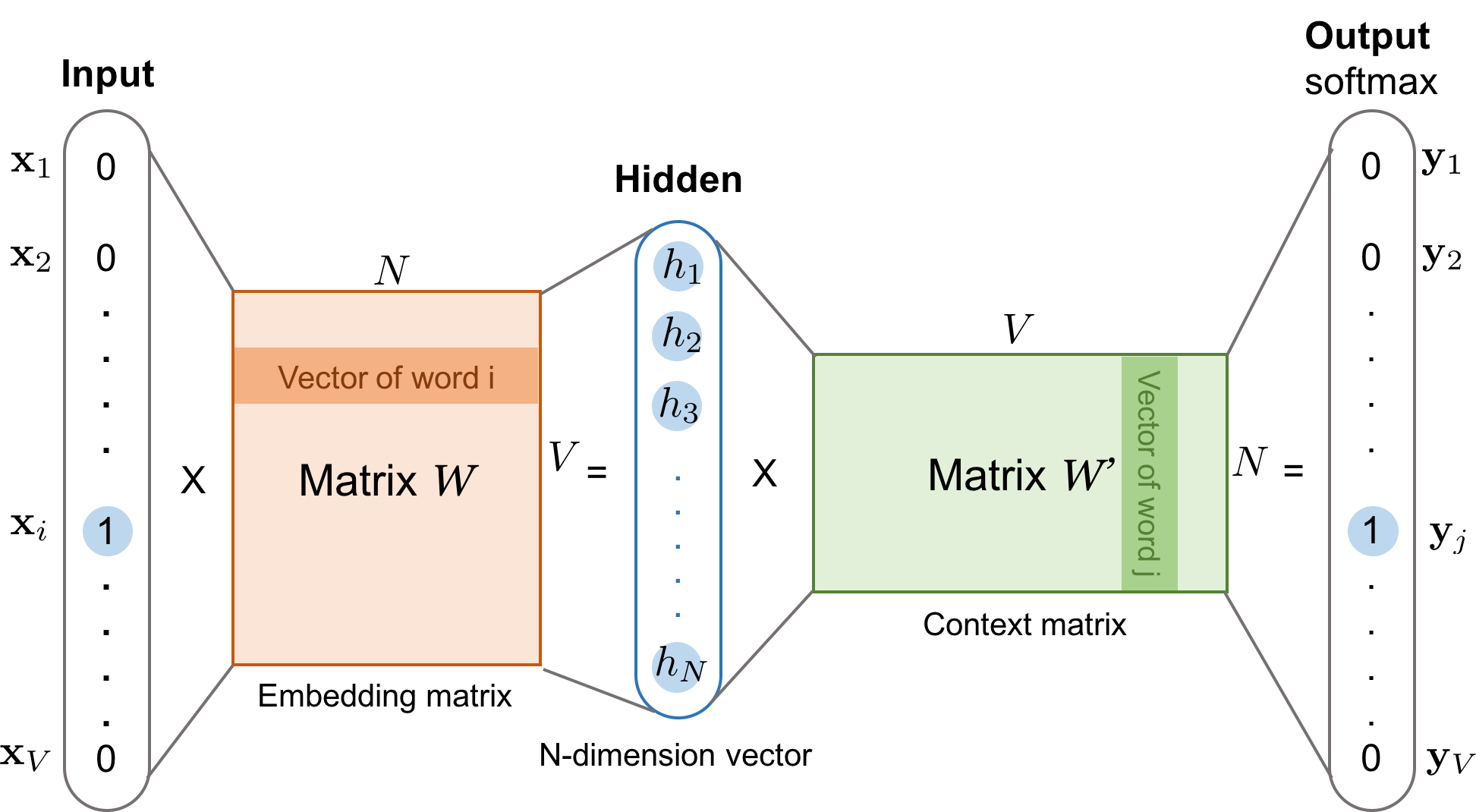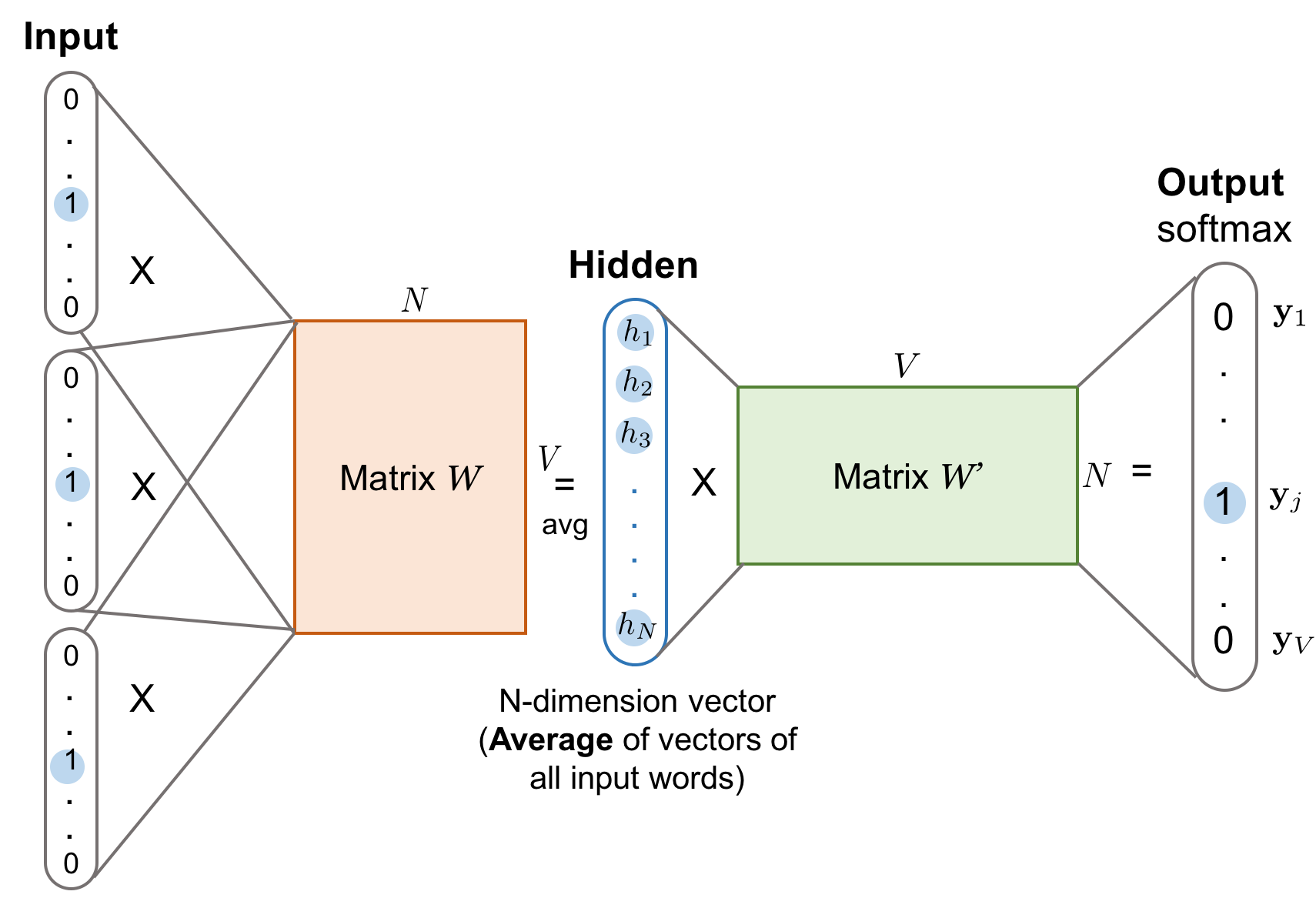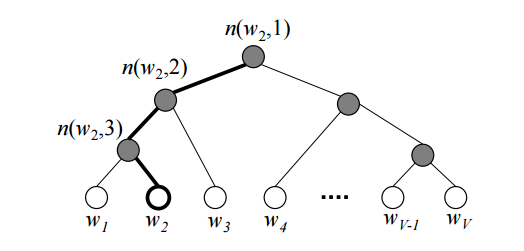Human vocabulary comes in free text. In order to make a machine learning model understand and process the natural language, we need to transform the free-text words into numeric values. One of the simplest transformation approaches is to do a one-hot encoding in which each distinct word stands for one dimension of the resulting vector and a binary value indicates whether the word presents (1) or not (0).

However, one-hot encoding is impractical computationally when dealing with the entire vocabulary, as the representation demands hundreds of thousands of dimensions. Word embedding represents words and phrases in vectors of (non-binary) numeric values with much lower and thus denser dimensions. An intuitive assumption for good word embedding is that they can approximate the similarity between words (i.e., “cat” and “kitten” are similar words, and thus they are expected to be close in the reduced vector space) or disclose hidden semantic relationships (i.e., the relationship between “cat” and “kitten” is an analogy to the one between “dog” and “puppy”). Contextual information is super useful for learning word meaning and relationship, as similar words may appear in the similar context often.

There are two main approaches for learning word embedding, both relying on the contextual knowledge.

• Count-based: The first one is unsupervised, based on matrix factorization of a global word co-occurrence matrix. Raw co-occurrence counts do not work well, so we want to do smart things on top.
• Context-based: The second approach is supervised. Given a local context, we want to design a model to predict the target words and in the meantime, this model learns the efficient word embedding representation.

# Count-Based Vector Space Model#

Count-based vector space models heavily rely on the word frequency and co-occurrence matrix with the assumption that words in the same contexts share similar or related semantic meanings. The models map count-based statistics like co-occurrences between neighboring words down to a small and dense word vectors. PCA, topic models, and neural probabilistic language models are all good examples of this category.

Different from the count-based approaches, context-based methods build predictive models that directly target at predicting a word given its neighbors. The dense word vectors are part of the model parameters. The best vector representation of each word is learned during the model training process.

# Context-Based: Skip-Gram Model#

Suppose that you have a sliding window of a fixed size moving along a sentence: the word in the middle is the “target” and those on its left and right within the sliding window are the context words. The skip-gram model (Mikolov et al., 2013) is trained to predict the probabilities of a word being a context word for the given target.

The following example demonstrates multiple pairs of target and context words as training samples, generated by a 5-word window sliding along the sentence.

“The man who passes the sentence should swing the sword.” – Ned Stark

Sliding window (size = 5) Target word Context
[The man who] the man, who
[The man who passes] man the, who, passes
[The man who passes the] who the, man, passes, the
[man who passes the sentence] passes man, who, the, sentence
[sentence should swing the sword] swing sentence, should, the, sword
[should swing the sword] the should, swing, sword
[swing the sword] sword swing, the
{:.info}

Each context-target pair is treated as a new observation in the data. For example, the target word “swing” in the above case produces four training samples: (“swing”, “sentence”), (“swing”, “should”), (“swing”, “the”), and (“swing”, “sword”).Given the vocabulary size $V$, we are about to learn word embedding vectors of size $N$. The model learns to predict one context word (output) using one target word (input) at a time.

According to Fig. 1,

• Both input word $w_i$ and the output word $w_j$ are one-hot encoded into binary vectors $\mathbf{x}$ and $\mathbf{y}$ of size $V$.
• First, the multiplication of the binary vector $\mathbf{x}$ and the word embedding matrix $W$ of size $V \times N$ gives us the embedding vector of the input word $w_i$: the i-th row of the matrix $W$.
• This newly discovered embedding vector of dimension $N$ forms the hidden layer.
• The multiplication of the hidden layer and the word context matrix $W’$ of size $N \times V$ produces the output one-hot encoded vector $\mathbf{y}$.
• The output context matrix $W’$ encodes the meanings of words as context, different from the embedding matrix $W$. NOTE: Despite the name, $W’$ is independent of $W$, not a transpose or inverse or whatsoever.

# Context-Based: Continuous Bag-of-Words (CBOW)#

The Continuous Bag-of-Words (CBOW) is another similar model for learning word vectors. It predicts the target word (i.e. “swing”) from source context words (i.e., “sentence should the sword”).Because there are multiple contextual words, we average their corresponding word vectors, constructed by the multiplication of the input vector and the matrix $W$. Because the averaging stage smoothes over a lot of the distributional information, some people believe the CBOW model is better for small dataset.

# Loss Functions#

Both the skip-gram model and the CBOW model should be trained to minimize a well-designed loss/objective function. There are several loss functions we can incorporate to train these language models. In the following discussion, we will use the skip-gram model as an example to describe how the loss is computed.

## Full Softmax#

The skip-gram model defines the embedding vector of every word by the matrix $W$ and the context vector by the output matrix $W’$. Given an input word $w_I$, let us label the corresponding row of $W$ as vector $v_{w_I}$ (embedding vector) and its corresponding column of $W’$ as $v’_{w_I}$ (context vector). The final output layer applies softmax to compute the probability of predicting the output word $w_O$ given $w_I$, and therefore:

$$p(w_O \vert w_I) = \frac{\exp({v'_{w_O}}^{\top} v_{w_I})}{\sum_{i=1}^V \exp({v'_{w_i}}^{\top} v_{w_I})}$$

This is accurate as presented in Fig. 1. However, when $V$ is extremely large, calculating the denominator by going through all the words for every single sample is computationally impractical. The demand for more efficient conditional probability estimation leads to the new methods like hierarchical softmax.

## Hierarchical Softmax#

Morin and Bengio (2005) proposed hierarchical softmax to make the sum calculation faster with the help of a binary tree structure. The hierarchical softmax encodes the language model’s output softmax layer into a tree hierarchy, where each leaf is one word and each internal node stands for relative probabilities of the children nodes.Each word $w_i$ has a unique path from the root down to its corresponding leaf. The probability of picking this word is equivalent to the probability of taking this path from the root down through the tree branches. Since we know the embedding vector $v_n$ of the internal node $n$, the probability of getting the word can be computed by the product of taking left or right turn at every internal node stop.

According to Fig. 3, the probability of one node is ($\sigma$ is the sigmoid function):

\begin{align} p(\text{turn right} \to \dots w_I \vert n) &= \sigma({v'_n}^{\top} v_{w_I})\\ p(\text{turn left } \to \dots w_I \vert n) &= 1 - p(\text{turn right} \vert n) = \sigma(-{v'_n}^{\top} v_{w_I}) \end{align}

The final probability of getting a context word $w_O$ given an input word $w_I$ is:

$$p(w_O \vert w_I) = \prod_{k=1}^{L(w_O)} \sigma(\mathbb{I}_{\text{turn}}(n(w_O, k), n(w_O, k+1)) \cdot {v'_{n(w_O, k)}}^{\top} v_{w_I})$$

where $L(w_O)$ is the depth of the path leading to the word $w_O$ and $\mathbb{I}_{\text{turn}}$ is a specially indicator function which returns 1 if $n(w_O, k+1)$ is the left child of $n(w_O, k)$ otherwise -1. The internal nodes’ embeddings are learned during the model training. The tree structure helps greatly reduce the complexity of the denominator estimation from O(V) (vocabulary size) to O(log V) (the depth of the tree) at the training time. However, at the prediction time, we still to compute the probability of every word and pick the best, as we don’t know which leaf to reach for in advance.

A good tree structure is crucial to the model performance. Several handy principles are: group words by frequency like what is implemented by Huffman tree for simple speedup; group similar words into same or close branches (i.e. use predefined word clusters, WordNet).

## Cross Entropy#

Another approach completely steers away from the softmax framework. Instead, the loss function measures the cross entropy between the predicted probabilities $p$ and the true binary labels $\mathbf{y}$.

First, let’s recall that the cross entropy between two distributions $p$ and $q$ is measured as $H(p, q) = -\sum_x p(x) \log q(x)$. In our case, the true label $y_i$ is 1 only when $w_i$ is the output word; $y_j$ is 0 otherwise. The loss function $\mathcal{L}_\theta$ of the model with parameter config $\theta$ aims to minimize the cross entropy between the prediction and the ground truth, as lower cross entropy indicates high similarity between two distributions.

$$\mathcal{L}_\theta = - \sum_{i=1}^V y_i \log p(w_i | w_I) = - \log p(w_O \vert w_I)$$

Recall that,

$$p(w_O \vert w_I) = \frac{\exp({v'_{w_O}}^{\top} v_{w_I})}{\sum_{i=1}^V \exp({v'_{w_i}}^{\top} v_{w_I})}$$

Therefore,

$$\mathcal{L}_{\theta} = - \log \frac{\exp({v'_{w_O}}^{\top}{v_{w_I}})}{\sum_{i=1}^V \exp({v'_{w_i}}^{\top}{v_{w_I} })} = - {v'_{w_O}}^{\top}{v_{w_I} } + \log \sum_{i=1}^V \exp({v'_{w_i} }^{\top}{v_{w_I}})$$

To start training the model using back-propagation with SGD, we need to compute the gradient of the loss function. For simplicity, let’s label $z_{IO} = {v’_{w_O}}^{\top}{v_{w_I}}$.

\begin{align} \nabla_\theta \mathcal{L}_{\theta} &= \nabla_\theta\big( - z_{IO} + \log \sum_{i=1}^V e^{z_{Ii}} \big) \\ &= - \nabla_\theta z_{IO} + \nabla_\theta \big( \log \sum_{i=1}^V e^{z_{Ii}} \big) \\ &= - \nabla_\theta z_{IO} + \frac{1}{\sum_{i=1}^V e^{z_{Ii}}} \sum_{i=1}^V e^{z_{Ii}} \nabla_\theta z_{Ii} \\ &= - \nabla_\theta z_{IO} + \sum_{i=1}^V \frac{e^{z_{Ii}}}{\sum_{i=1}^V e^{z_{Ii}}} \nabla_\theta z_{Ii} \\ &= - \nabla_\theta z_{IO} + \sum_{i=1}^V p(w_i \vert w_I) \nabla_\theta z_{Ii} \\ &= - \nabla_\theta z_{IO} + \mathbb{E}_{w_i \sim Q(\tilde{w})} \nabla_\theta z_{Ii} \end{align}

where $Q(\tilde{w})$ is the distribution of noise samples.

According to the formula above, the correct output word has a positive reinforcement according to the first term (the larger $\nabla_\theta z_{IO}$ the better loss we have), while other words have a negative impact as captured by the second term.

How to estimate $\mathbb{E}_{w_i \sim Q(\tilde{w})} \nabla_\theta {v’_{w_i}}^{\top}{v_{w_I}}$ with a sample set of noise words rather than scanning through the entire vocabulary is the key of using cross-entropy-based sampling approach.

## Noise Contrastive Estimation (NCE)#

The Noise Contrastive Estimation (NCE) metric intends to differentiate the target word from noise samples using a logistic regression classifier (Gutmann and Hyvärinen, 2010).

# Examples: word2vec on “Game of Thrones”#

After reviewing all the theoretical knowledge above, let’s try a little experiment in word embedding extracted from “the Games of Thrones corpus”. The process is super straightforward using gensim.

Step 1: Extract words

import sys
from nltk.corpus import stopwords
from nltk.tokenize import sent_tokenize

STOP_WORDS = set(stopwords.words('english'))

def get_words(txt):
return filter(
lambda x: x not in STOP_WORDS,
re.findall(r'\b(\w+)\b', txt)
)

def parse_sentence_words(input_file_names):
"""Returns a list of a list of words. Each sublist is a sentence."""
sentence_words = []
for file_name in input_file_names:
for line in open(file_name):
line = line.strip().lower()
line = line.decode('unicode_escape').encode('ascii','ignore')
sent_words = map(get_words, sent_tokenize(line))
sent_words = filter(lambda sw: len(sw) > 1, sent_words)
if len(sent_words) > 1:
sentence_words += sent_words
return sentence_words

# You would see five .txt files after unzip 'a_song_of_ice_and_fire.zip'
input_file_names = ["001ssb.txt", "002ssb.txt", "003ssb.txt",
"004ssb.txt", "005ssb.txt"]
GOT_SENTENCE_WORDS= parse_sentence_words(input_file_names)


Step 2: Feed a word2vec model

from gensim.models import Word2Vec

# size: the dimensionality of the embedding vectors.
# window: the maximum distance between the current and predicted word within a sentence.
model = Word2Vec(GOT_SENTENCE_WORDS, size=128, window=3, min_count=5, workers=4)
model.wv.save_word2vec_format("got_word2vec.txt", binary=False)


Step 3: Check the results

In the GoT word embedding space, the top similar words to “king” and “queen” are:

model.most_similar('king', topn=10)
(word, similarity with ‘king’)
model.most_similar('queen', topn=10)
(word, similarity with ‘queen’)
(‘kings’, 0.897245) (‘cersei’, 0.942618)
(‘baratheon’, 0.809675) (‘joffrey’, 0.933756)
(‘son’, 0.763614) (‘margaery’, 0.931099)
(‘robert’, 0.708522) (‘sister’, 0.928902)
(’lords’, 0.698684) (‘prince’, 0.927364)
(‘joffrey’, 0.696455) (‘uncle’, 0.922507)
(‘prince’, 0.695699) (‘varys’, 0.918421)
(‘brother’, 0.685239) (’ned’, 0.917492)
(‘aerys’, 0.684527) (‘melisandre’, 0.915403)
(‘stannis’, 0.682932) (‘robb’, 0.915272)

Cited as:

@article{weng2017wordembedding,
title   = "Learning word embedding",
author  = "Weng, Lilian",
journal = "lilianweng.github.io",
year    = "2017",
url     = "https://lilianweng.github.io/posts/2017-10-15-word-embedding/"
}


 Tensorflow Tutorial Vector Representations of Words.

 “Word2Vec Tutorial - The Skip-Gram Model” by Chris McCormick.

 “On word embeddings - Part 2: Approximating the Softmax” by Sebastian Ruder.

 Xin Rong. word2vec Parameter Learning Explained

 Mikolov, Tomas, Kai Chen, Greg Corrado, and Jeffrey Dean. “Efficient estimation of word representations in vector space.” arXiv preprint arXiv:1301.3781 (2013).

 Frederic Morin and Yoshua Bengio. “Hierarchical Probabilistic Neural Network Language Model.” Aistats. Vol. 5. 2005.

 Michael Gutmann and Aapo Hyvärinen. “Noise-contrastive estimation: A new estimation principle for unnormalized statistical models.” Proc. Intl. Conf. on Artificial Intelligence and Statistics. 2010.

 Tomas Mikolov, Ilya Sutskever, Kai Chen, Greg Corrado, and Jeffrey Dean. “Distributed representations of words and phrases and their compositionality.” Advances in neural information processing systems. 2013.

 Tomas Mikolov, Kai Chen, Greg Corrado, and Jeffrey Dean. “Efficient estimation of word representations in vector space.” arXiv preprint arXiv:1301.3781 (2013).

 Marco Baroni, Georgiana Dinu, and Germán Kruszewski. “Don’t count, predict! A systematic comparison of context-counting vs. context-predicting semantic vectors.” ACL (1). 2014.

 Jeffrey Pennington, Richard Socher, and Christopher Manning. “Glove: Global vectors for word representation.” Proc. Conf. on empirical methods in natural language processing (EMNLP). 2014.